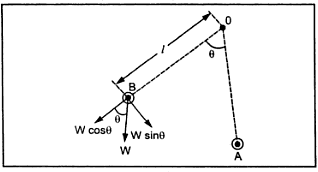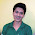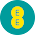Controlling System

This system should provided a force so that current or any other electrical quantity will produce deflection of the pointer proportional to its magnitude. The important functions of this system are,
1) It produces a force equal and opposite to the deflecting force in order to make the deflection of pointer at a definite magnitude. If this system is absent, then the pointer will swing beyond its final steady positions for the given magnitude and deflection will become indefinite.
2) It brings the moving system back to zero position when the force which causes the movement of the moving system is removed. It will never come back to its zero position in the absence of controlling system.
Controlling torque is generally provided by springs. Sometimes gravity control is also used.
1.1 Gravity Control
This type of control consists of a small wight attached to the moving system whose position is adjustable. This weight produces a controlling torque due to gravity. This weight is called control weight.
The Fig. 1 shows the gravity control system. At the zero position of the pointer, the controlling torque is zero. This position is shown as position A of the weight in the Fig. 2. if the system deflects, the weight positions also changes, as shown in the Fig.2.Fig. 1 Gravity Control

The system deflects through an angle θ. The control weight acts at a distance l  from the center. The component Wsinθ of this weight tries to restore the pointer back to the zero position. This is nothing but the controlling torque Tc.Fig. 2

Thus,
controlling torque T= Wsinθ x
= Ksin θ
here             K = W
= gravity constant
Now generally all meters are current sensing meters where,
deflecting torque T= Kt  I
where Kt= another constant.
In equilibrium position, Td  = Tc
...          Kt  I = Ksinθ
...          I  α  sinθ
Thus the deflection is proportional to current i.e. quantity to be measured. But as it is a function of sinθ , the scale for the instrument using gravity control is not uniform.
1) Its performance is not time dependent.
2) It is simple and cheap.
3) The controlling torque can be varied by adjusting the position of the control weight.
4) Its performance is not temperature dependent.
1) The scale is nonuniform causing problems to record accurate readings.
2) The system must be used in vertical position only and must be properly levelled.
Otherwise it may cause serious errors in the measurement.
3) As delicate and proper levelling required, in general it is not used for indicating instruments and portable instruments.
1.2 Spring Control
Two hair springs are attached to the moving system which exerts controlling torque. To employ spring control to an instrument, following requirements are essential.
1) The spring should be non-magnetic.
2) The spring should be free from mechanical stress.
3) The spring should have a small resistance, sufficient cross-sectional area.
4) It should have low resistance temperature co-efficient.
The arrangement of the springs is shown in the Fig.3Fig. 3 Spring Control

The springs are made up of non-magnetic materials like silicon bronze, hard rolled silver copper, platinum silver and german silver. For most of the instruments, phosphor bronze spiral are provided. Flat spiral springs are used in almost all indicating instruments.
The inner of the spring is attached to the spindle while the outer end is attached to a lever or arm which is actuated by a set of screw mounted at the front of the instrument. So zero setting can be easily done. The controlling torque provided by the instrument is directly proportional to the angular deflection of the pointer.
The controlling torque produced by spiral springs is given by,

Now deflecting torque is proportional to current.
...       Td  α  I
At equilibrium,    Td  = Tc
...         I  α  θ
Thus the deflection is proportional to the current. Hence the scale of the instrument using spring control is uniform. When the current is removed, due to spring force the pointer comes back to initial positions. The spring control is very popular and is used in almost all indicating instruments.

1.3 Comparison of Controlling Systems

1.2.